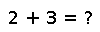# set_zero_subnormals

set_zero_subnormals(yes::Bool) -> Bool

If `yes` is `false`, subsequent floating-point operations follow rules for IEEE arithmetic on subnormal values ("denormals"). Otherwise, floating-point operations are permitted (but not required) to convert subnormal inputs or outputs to zero. Returns `true` unless `yes==true` but the hardware does not support zeroing of subnormal numbers.

`set_zero_subnormals(true)` can speed up some computations on some hardware. However, it can break identities such as `(x-y==0) == (x==y)`.

## Examples

Checking you are not a robot: